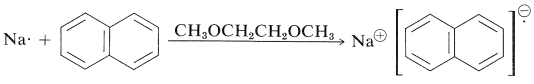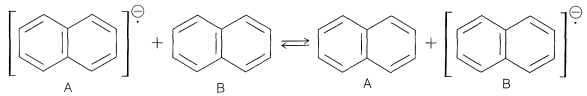# 27.10: Electron-Spin Resonance (ESR) Spectroscopy of Organic Radicals

$$\newcommand{\vecs}{\overset { \rightharpoonup} {\mathbf{#1}} }$$ $$\newcommand{\vecd}{\overset{-\!-\!\rightharpoonup}{\vphantom{a}\smash {#1}}}$$$$\newcommand{\id}{\mathrm{id}}$$ $$\newcommand{\Span}{\mathrm{span}}$$ $$\newcommand{\kernel}{\mathrm{null}\,}$$ $$\newcommand{\range}{\mathrm{range}\,}$$ $$\newcommand{\RealPart}{\mathrm{Re}}$$ $$\newcommand{\ImaginaryPart}{\mathrm{Im}}$$ $$\newcommand{\Argument}{\mathrm{Arg}}$$ $$\newcommand{\norm}{\| #1 \|}$$ $$\newcommand{\inner}{\langle #1, #2 \rangle}$$ $$\newcommand{\Span}{\mathrm{span}}$$ $$\newcommand{\id}{\mathrm{id}}$$ $$\newcommand{\Span}{\mathrm{span}}$$ $$\newcommand{\kernel}{\mathrm{null}\,}$$ $$\newcommand{\range}{\mathrm{range}\,}$$ $$\newcommand{\RealPart}{\mathrm{Re}}$$ $$\newcommand{\ImaginaryPart}{\mathrm{Im}}$$ $$\newcommand{\Argument}{\mathrm{Arg}}$$ $$\newcommand{\norm}{\| #1 \|}$$ $$\newcommand{\inner}{\langle #1, #2 \rangle}$$ $$\newcommand{\Span}{\mathrm{span}}$$

An important method of studying radicals is electron-spin resonance (ESR) spectroscopy. The principles of this form of spectroscopy are much the same as of NMR spectroscopy, but the language used by the practitioners of these two forms of magnetic resonance spectroscopy is different.

First, let us discuss the similarities. The important point is that an unpaired electron, like a proton, has a spin and a magnetic moment such that it has two possible orientations in a magnetic field. The two orientations correspond to magnetic quantum numbers $$+\frac{1}{2}$$ and $$-\frac{1}{2}$$ that define two energy states. These states differ in energy by $$\Delta E = \left( h \gamma \right) H$$, in which $$\gamma$$ is the gyromagnetic ratio of the electron. (See Section 9-10A for a discussion of the analogous situation for protons.) Transitions between these states occur with absorption of radiation of frequency $$\nu = \gamma H$$. Because $$\gamma$$ for free electrons is about 1000 times larger than $$\gamma$$ for protons, the frequency of absorption $$nu$$ of electrons is about 1000 times that of protons at the same magnetic field. At magnetic fields of 3600 gauss the absorption frequency of free electrons is about $$10,000 \: \text{MHz}$$, which falls in the microwave, rather than the radio-wave region.

The basic apparatus for ESR spectroscopy is similar to that shown in Figure 9-22 for NMR spectroscopy, except that the sample is irradiated with a microwave generator. The spectra produced by ESR absorptions of unpaired electrons are similar to those shown in Figure 9-25, except that ESR spectrometers normally are so arranged as to yield a plot of the first derivative of the curve of absorption against magnetic field rather than the absorption curve itself, as shown in Figure 27-17. This arrangement is used because it gives a better signal-to-noise ratio than a simple plot of absorption against magnetic field.Figure 27-17: Plots of (a) absorption and (b) derivative ESR curves.

The sensitivity of ESR spectroscopy for detection of radicals is very high. Under favorable conditions, a concentration of radicals as low as $$10^{-12} \: \text{M}$$ can be detected readily. Identification of simple hydrocarbon radicals often is possible by analysis of the fine structure in their spectra, which arises from spin-spin splittings involving those protons that are reasonably close to the centers over which the unpaired electron is distributed. Methyl radicals, $$\ce{CH_3} \cdot$$, generated by x-ray bombardment of methyl iodide at $$-196^\text{o}$$ show four resonance lines of intensity 1:3:3:1, as expected for interaction of the electron with $$n + 1$$ protons (see Section 9-10G).

The chemical shift generally is much less important in ESR spectroscopy than in NMR. One reason is that the lifetimes of the electrons in the $$+\frac{1}{2}$$ and $$-\frac{1}{2}$$ states generally are very short ($$10^{-6} \: \text{sec}$$ or less) so ESR lines are quite broad by comparison with NMR lines (Section 27-1). ESR chemical shifts usually are measured in terms of "$$g$$ factors", which, like NMR $$\delta$$ values, are field-independent. The resonance frequency is given by $$\nu = g \mu_0 H_0/h$$, in which $$\mu_0$$ is the magnetic moment of the electron.

Spin-spin splittings arising from proton-electron interactions are very large in ESR spectra and usually are reported in gauss, under the heading hyperfine interactions. The proton-electron splitting in the methyl radical is 23 gauss $$\left( 64.4 \: \text{MHz} \right)$$, which is vastly larger than the $$7$$-$$\text{Hz}$$ proton-proton splitting in ethanol (Figure 9-23). The large splittings (and broad lines) typical of ESR make it possible to run ESR spectra on solids or highly viscous materials, for which the fine structure typical of high-resolution NMR spectra would be wholly washed out (Section 27-1).

ESR spectra are subject to exchange effects in the same way as NMR spectra. A specific example is provided by electron exchange between sodium naphthalenide and naphthalene. Naphthalene has a set of ten $$\pi$$-molecular orbitals, similar to the six $$\pi$$-molecular orbitals of benzene (Figure 21-5). The ten naphthalene $$\pi$$ electrons fill the lower five of these orbitals. In a solvent such as 1,2-dimethoxyethane, which solvates small metal ions well, naphthalene accepts an electron from a sodium atom and forms sodium naphthalenide, a radical anion:The additional electron goes into the lowest unoccupied molecular orbital of the napthalene, which means the electron circulates over all of the carbons. The electron resonance is split into a total of 25 lines by electron-proton magnetic interactions. The reason for the complex splitting can be understood if we notice that there are eight protons in two sets of four. One set spits the electron signal into five lines $$\left( n + 1 \right)$$ of intensity 1:4:6:4:1 with a spacing of 5.0 gauss, while the second set splits each of the five lines into another 1:4:6:4:1 quintet with a spacing of 1.9 gauss. So, in all, there are twenty-five lines - five sets of five.

If excess naphthalene is added to a solution of sodium naphthalenide, intermolecular electron exchange occurs:This means that the electron goes from naphthalene A with a particular set of $$+\frac{1}{2}, -\frac{1}{2}$$ proton nuclei to naphthalene B with a different set. The result is that the lines broaden and, if the exchange is very fast, the splitting vanishes. Because the splittings area bout 5 gauss $$\left( 14 \: \text{MHz} \right)$$, the mean lifetime before exchange has to be about $$10^{-8} \: \text{sec}$$ or less to obscure the splitting (see Sections 27-1 and 27-2).

The most exciting applications of ESR are in the study of radical intermediates in organic reactions. Considerable use has been made of the technique in biochemical reactions and it has been shown that radicals are generated and decay in oxidations brought about by enzymes. Radicals also have been detected by ESR measurements in algae that "fix" carbon dioxide in photosynthesis. The character of the radicals formed has been found to depend upon the wavelength of the light supplied for photosynthesis.